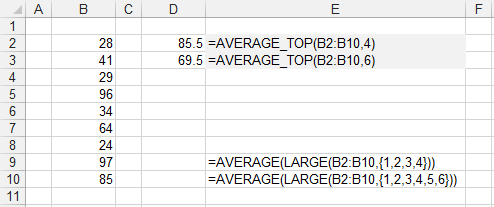# AVERAGETOP

There are 2 functions for getting the average.

### Remarks

* For instructions on how to add a function to a workbook refer to the page under Inserting Functions

### AVERAGETOP

Returns the average from a range of values only considering the top "x" values

`'rgeAverageRange - The range of values you want to average.'iTop - The value indicating the top number.Public Function AVERAGE_TOP(ByVal rgeCriteria As Range, _                             ByVal iTop As Integer) As Single Dim inumber As Integer Dim sngAverage As Single     Call Application.Volatile(True)         If (iTop > rgeCriteria.Cells.Count) Then         AVERAGE_TOP = 0         Exit Function     End If         sngAverage = 0     For inumber = 1 To iTop         sngAverage = sngAverage + Application.WorksheetFunction.Large(rgeCriteria, inumber)     Next inumber             AVERAGE_TOP = sngAverage / iTop End Function `

If iTop is greater than the number of items then 0 is returned.### AVERAGETOP_PERCENT

Returns the average of the visible, non blank cells, only considering the top "x" percent.